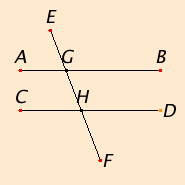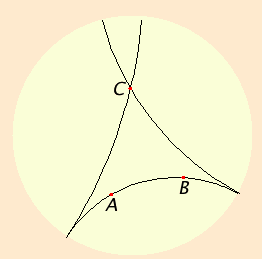# Proposition 29

A straight line falling on parallel straight lines makes the alternate angles equal to one another, the exterior angle equal to the interior and opposite angle, and the sum of the interior angles on the same side equal to two right angles.

Let the straight line EF fall on the parallel straight lines AB and CD.

I say that it makes the alternate angles AGH and GHD equal, the exterior angle EGB equal to the interior and opposite angle GHD, and the sum of the interior angles on the same side, namely BGH and GHD, equal to two right angles.If the angle AGH does not equal the angle GHD, then one of them is greater. Let the angle AGH be greater.

Add the angle BGH to each. Therefore the sum of the angles AGH and BGH is greater than the sum of the angles BGH and GHD.

I.13

But sum of the angles AGH and BGH equals two right angles. Therefore the sum of the angles BGH and GHD is less than two right angles.

Post.5

But straight lines produced indefinitely from angles less than two right angles meet. Therefore AB and CD, if produced indefinitely, will meet. But they do not meet, because they are by hypothesis parallel.

Therefore the angle AGH is not unequal to the angle GHD, and therefore equals it.

Again, the angle AGH equals the angle EGB. Therefore the angle EGB also equals the angle GHD.

C.N.2

Add the angle BGH to each. Therefore the sum of the angles EGB and BGH equals the sum of the angles BGH and GHD.

But the sum of the angles EGB and BGH equals two right angles. Therefore the sum of the angles BGH and GHD also equals two right angles.

Therefore a straight line falling on parallel straight lines makes the alternate angles equal to one another, the exterior angle equal to the interior and opposite angle, and the sum of the interior angles on the same side equal to two right angles.

Q.E.D.

## Guide

The statement of this proposition includes three parts, one the converse of I.27, the other two the converse of I.28. Like those propositions, this one assumes an ambient plane containing all the three lines.

This is the first proposition which depends on the parallel postulate. As such it does not hold in hyperbolic geometry.

#### Hyperbolic geometry

Two important geometries alternative to Euclidean geometry are elliptic geometry and hyperbolic geometry. Elliptic geometry was discussed in the note after Proposition I.16, that being the first proposition which doesn’t hold in elliptic geometry. This, I.29, is the first which doesn’t hold in hyperbolic geometry.

These three geometries can be distinguished by the number of lines parallel to a given line passing through a given point. For elliptic geometry, there is no such parallel line; for Euclidean geometry (which may be called parabolic geometry), there is exactly one; and for hyperbolic geometry, there are infinitely many.

It is not possible to illustrate hyperbolic geometry with correct distances on a flat surface since a flat surface is Euclidean. Poincaré, however, described a useful model of hyperbolic geometry where the “points” in a hyperbolic plane are taken to be points inside a fixed circle (but not the points on the circumference). The “lines” in the hyperbolic plane are the parts of circles orthogonal, that is, at right angles to the fixed circle. And in this model, “angles” in the hyperbolic plane are angles between these arcs, or, more precisely, angles between the tangents to the arcs at the point of intersection. Since “angles” are just angles, this model is called a conformal model. Distances in the hyperbolic plane, however, are not measured by distances along the arcs. There is a more complicated relation between distances so that near the edge of the fixed circle a very short arc models a very long “line.”

 Once this model is accepted, it is easy to see why there are infinitely many “lines” parallel to a given “line” through a given “point.” That is just that there are infinitely many circles orthogonal to the fixed circle which don’t intersect the given circle orthogonal to the fixed circle but do pass through the given point. In the diagram, AB is a “line” in the hyperbolic plane, that is, a circle orthogonal to the circumference of the shaded disk which represents the hyperbolic plane. A “point” C lies in that plane. Two “lines” are shown passing through C, one gets close to the line AB in the direction of A, the other gets close in the direction of B. But these two “lines” don’t intersect AB since the arcs representing them only intersect on the circumference of the disk, and points on the circumference don’t represent “points” in the hyperbolic plane.These two parallel “lines” are called the asymptotic parallels of AB since they approach AB at one end or the other. There are infinitely many parallels between them. (In much of the literature on hyperbolic geometry, the word “parallels” is used for what are called “asymptotic parallels” here, while “nonintersecting lines” is used for what are called “parallels” here.)

#### Use of Proposition 29

This proposition is used in very frequently in Book I starting with the next proposition. It is also used frequently in Book II, VI, and XI, and once in Book XII.